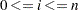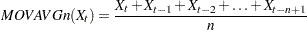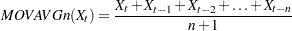Functions across Time

PROC MODEL provides four types of special built-in functions that refer to the values of variables and expressions in previous time periods. These functions have the following forms where n represents the number of periods, x is any expression, and the argument i is a variable or expression that gives the lag length (). If the index value i is omitted, the maximum lag length n is used.

LAGn ( < i,> x )

returns the ith lag of x, where n is the maximum lag;

DIFn (x )

is the difference of x at lag n

ZLAGn ( < i,> x )

returns the ith lag of x, where n is the maximum lag, with missing lags replaced with zero

XLAGn ( x, y )

returns the nth lag of x if x is nonmissing, or y if x is missing

ZDIFn (x )

is the difference with lag length truncated and missing values converted to zero; x is the variable or expression to compute the moving average of

MOVAVGn( x )

is the moving average if Xdenotes the observation at time point t, to ensure compatibility with the number n of observations used to calculate the moving average MOVAVGn, the following definition is used:The moving average calculation for SAS 9.1 and earlier releases is as follows:Missing values of x are omitted in computing the average.

If you do not specify n, the number of periods is assumed to be one. For example, LAG(X) is the same as LAG1(X). No more than four digits can be used with a lagging function; that is, LAG9999 is the greatest LAG function, ZDIF9999 is the greatest ZDIF function, and so on.

The LAG functions get values from previous observations and make them available to the program. For example, LAG(X) returns the value of the variable X as it was computed in the execution of the program for the preceding observation. The expression LAG2(X+2*Y) returns the value of the expression X+2*Y, computed by using the values of the variables X and Y that were computed by the execution of the program for the observation two periods ago.

The DIF functions return the difference between the current value of a variable or expression and the value of its LAG. For example, DIF2(X) is a short way of writing X–LAG2(X), and DIF15(SQRT(2*Z)) is a short way of writing SQRT(2*Z)–LAG15(SQRT(2*Z)).

The ZLAG and ZDIF functions are like the LAG and DIF functions, but they are not counted in the determination of the program lag length, and they replace missing values with 0s. The ZLAG function returns the lagged value if the lagged value is nonmissing, or 0 if the lagged value is missing. The ZDIF function returns the differenced value if the differenced value is nonmissing, or 0 if the value of the differenced value is missing. The ZLAG function is especially useful for models with ARMA error processes. See the next section for details.

Lag Logic

The LAG and DIF lagging functions in the MODEL procedure are different from the queuing functions with the same names in the DATA step. Lags are determined by the final values that are set for the program variables by the execution of the model program for the observation. This can have upsetting consequences for programs that take lags of program variables that are given different values at various places in the program, as shown in the following statements:

```   temp = x + w;
t    = lag( temp );
temp = q - r;
s    = lag( temp );
```

The expression LAG(TEMP) always refers to LAG(Q–R), never to LAG(X+W), since Q–R is the final value assigned to the variable TEMP by the model program. If LAG(X+W) is wanted for T, it should be computed as T=LAG(X+W) and not T=LAG(TEMP), as in the preceding example.

Care should also be exercised in using the DIF functions with program variables that might be reassigned later in the program. For example, the program

```   temp =  x ;
s    = dif( temp );
temp = 3 * y;
```

computes values for S equivalent to

```   s =  x  - lag( 3 * y );
```

Note that in the preceding examples, TEMP is a program variable, not a model variable. If it were a model variable, the assignments to it would be changed to assignments to a corresponding equation variable.

Note that whereas LAG1(LAG1(X)) is the same as LAG2(X), DIF1(DIF1(X)) is not the same as DIF2(X). The DIF2 function is the difference between the current period value at the point in the program where the function is executed and the final value at the end of execution two periods ago; DIF2 is not the second difference. In contrast, DIF1(DIF1(X)) is equal to DIF1(X)-LAG1(DIF1(X)), which equals X–2*LAG1(X)+LAG2(X), which is the second difference of X.

Lag Lengths

The lag length of the model program is the number of lags needed for any relevant equation. The program lag length controls the number of observations used to initialize the lags.

PROC MODEL keeps track of the use of lags in the model program and automatically determines the lag length of each equation and of the model as a whole. PROC MODEL sets the program lag length to the maximum number of lags needed to compute any equation to be estimated, solved, or needed to compute any instrument variable used.

In determining the lag length, the ZLAG and ZDIF functions are treated as always having a lag length of 0. For example, if Y is computed as

```   y = lag2( x + zdif3( temp ) );
```

then Y has a lag length of 2 (regardless of how TEMP is defined). If Y is computed as

```   y = zlag2( x + dif3( temp ) );
```

then Y has a lag length of 0.

This is so that ARMA errors can be specified without causing the loss of additional observations to the lag starting phase and so that recursive lag specifications, such as moving-average error terms, can be used. Recursive lags are not permitted unless the ZLAG or ZDIF functions are used to truncate the lag length. For example, the following statement produces an error message:

```   t = a + b * lag( t );
```

The program variable T depends recursively on its own lag, and the lag length of T is therefore undefined.

In the following equation RESID.Y depends on the predicted value for the Y equation but the predicted value for the Y equation depends on the LAG of RESID.Y, and thus, the predicted value for the Y equation depends recursively on its own lag.

```   y = yhat + ma * lag( resid.y );
```

The lag length is infinite, and PROC MODEL prints an error message and stops. Since this kind of specification is allowed, the recursion must be truncated at some point. The ZLAG and ZDIF functions do this.

The following equation is valid and results in a lag length for the Y equation equal to the lag length of YHAT:

```   y = yhat + ma * zlag( resid.y );
```

Initially, the lags of RESID.Y are missing, and the ZLAG function replaces the missing residuals with 0s, their unconditional expected values.

The ZLAG0 function can be used to zero out the lag length of an expression. ZLAG0(x ) returns the current period value of the expression x, if nonmissing, or else returns 0, and prevents the lag length of x from contributing to the lag length of the current statement.

Initializing Lags

At the start of each pass through the data set or BY group, the lag variables are set to missing values and an initialization is performed to fill the lags. During this phase, observations are read from the data set, and the model variables are given values from the data. If necessary, the model is executed to assign values to program variables that are used in lagging functions. The results for variables used in lag functions are saved. These observations are not included in the estimation or solution.

If, during the execution of the program for the lag starting phase, a lag function refers to lags that are missing, the lag function returns missing. Execution errors that occur while starting the lags are not reported unless requested. The modeling system automatically determines whether the program needs to be executed during the lag starting phase.

If L is the maximum lag length of any equation being fit or solved, then the first L observations are used to prime the lags. If a BY statement is used, the first L observations in the BY group are used to prime the lags. If a RANGE statement is used, the first L observations prior to the first observation requested in the RANGE statement are used to prime the lags. Therefore, there should be at least L observations in the data set.

Initial values for the lags of model variables can also be supplied in VAR, ENDOGENOUS, and EXOGENOUS statements. This feature provides initial lags of solution variables for dynamic solution when initial values for the solution variable are not available in the input data set. For example, the statement

```   var x 2 3 y 4 5 z 1;
```

feeds the initial lags exactly like these values in an input data set:

Lag

X

Y

Z

2

3

5

.

1

2

4

1

If initial values for lags are available in the input data set and initial lag values are also given in a declaration statement, the values in the VAR, ENDOGENOUS, or EXOGENOUS statements take priority.

The RANGE statement is used to control the range of observations in the input data set that are processed by PROC MODEL. In the following statement, ‘01jan1924’ specifies the starting period of the range, and ‘01dec1943’ specifies the ending period:

```   range date = '01jan1924'd to '01dec1943'd;
```

The observations in the data set immediately prior to the start of the range are used to initialize the lags.